### Fundamental Property Relations

Fundamental thermodynamic property relations for a fluid of constant composition are as follows: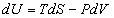→ I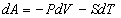→ II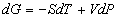→ III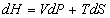→ IV

Criterion of exactness for a differential expression:

If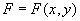, then the total differnential of F is defined as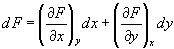→ V

Otherwise,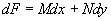where,and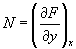By further differentiation, we obtain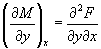and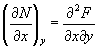Since the order of differentiation in mixed second derivatives is immaterial, these equations give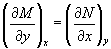→ VI